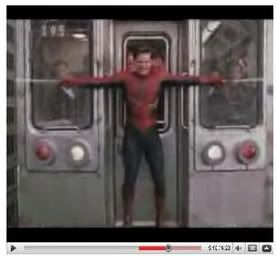### Spider-Man 2

 "Spider-Man Train Stopping" Scene Is it possible?   By: Katie PolakowskiIn the movie Spider-Man 2, there is a scene which shows spiderman stopping a moving train before falling off the edge of broken tracks. When attempting to stop the train with his foot does not work, he decides to attach his webs to near building. At first he only uses two webs. When this doesn't work, Spider-Man attaches multiple webs to building all around him. This successfully slows the train just in time in order to save the passengers.   The main question is "If this were possible, what would the tension of Spider-Man's webs be?"     Here is the link to view the "Train Scene" http://www.youtube.com/watch?v=GYOYewO_Veg&feature=related                   Free Body Diagram (click to view image) How to find the tension of Spider-Man's web necessary to stop moving train:   Initial speed of train = 35.76 m/s (80 mph)                 I figured out the speed of the train (which equals 80mph) by viewing the movie. In one of the scenes, it is shown that the train reaches 80mph after Doc Ock desroys the break on the train.                     1mph = 0.44704mps                      80(0.44704) = 35.7632                                        = 35.76m/s     vf = 0m/s     vi = 35.76m/s Mass of train = approximately 137,100 kg (302,254 lbs)  (found in research) Time taken to stop train = approximately 46 seconds     a = vf - vi         t    = 0 - 35.76           46    = -.7773913043    = -.78 m/s^2  (The number is negative since the accelerarion of the train is slowing down)    According to Newton's Second Law, a = f/m. When rearranged, the formula reads f = m(a). As shown in the free body diagram, the weight of the train equals the force due to gravity.   (force = tension) F =  m(a)    = 137,100(-.78)    = -106938 N   106938 N is the calculated tension of Spider-Man's webs   I also calculated the distance it took for Spider-Man to stop the train: Approximate distance traveled by train: d = 1/2(a)(t)^2    = 1/2(-.78)(46)^2    = 825.24 m          In order for Spider-Man to have been able to completely stop the train before falling off the edge of the tracks, I found that the tension of his webs must have equaled 106938N.       In order to determine the tension of Spider-Man's webs, I needed to know the mass of the train. Since the movie was unclear on what type of trian was used, I researched online to find the mass of the train. Knowing the mass of the train, i was then able to calculate the tension of Spider-Man's webs and the distance the train took to stop.   Works Cited "Spider Man (2002)." Hypertextbook.com. Web. 03 Jan. 2010. .